Time: 3 Hours                                                                                                     Max. Marks: 100

NOTE: There are 9 Questions in all.

·      Question 1 is compulsory and carries 20 marks. Answer to Q. 1. must be written in the space provided for it in the answer book supplied and nowhere else.

·      Out of the remaining EIGHT Questions answer any FIVE Questions. Each question carries 16 marks.

·      Any required data not explicitly given, may be suitably assumed and stated.

Q.1       Choose the correct or best alternative in the following:                                         (2x10)

a.       The number 140 in octal is equivalent to

(A)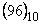.                                        (B)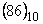.

(C)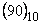.                                        (D)  none of these.

b.      The NOR gate output will be low if the two inputs are

(A)    00                                                (B)  01

(C)  10                                                (D)  11

c.   Which of the following is the fastest logic?

(A)    ECL                                             (B)  TTL

(C)  CMOS                                        (D)  LSI

d.   How many flipflops are required to construct mod 30 counter

(A)  5                                                  (B)  6

(C)  4                                                  (D)  8

e.   How many address bits are required to represent a 32 K memory

(A)     10 bits.                                        (B)  12 bits.

(C)  14 bits.                                         (D)  16 bits.

f.    The number of control lines for 16 to 1 multiplexer is

(A)     2.                                                 (B)  4.

(C)  3.                                                 (D)  5.

g.   For 10 bit ADC, full scale input is 10 V.  The resolution is

(A) 10 mV.                                         (B)  0 mV.

(C)  9.74 mV.                                     (D)  none of these.

h.   Which of following requires refreshing?

(A)    SRAM.                                        (B)  DRAM.

(C)  ROM.                                          (D)  EPROM.

i.    Shifting a register content to left by one bit position is equivalent to

(A)   division by two.                            (B) addition by two.

(C) multiplication by two.                     (D) subtraction by two.

j.    For JK flip flop with J=1, K=0, the output after clock pulse will be

(A)  0.                                                 (B)  1.

(C)  high impedance.                            (D)  no change.

Answer any FIVE Questions out of EIGHT Questions.

Each question carries 16 marks.

Q.2     a.   Perform following subtraction using 1’s complement

(i)  11001 – 10110                              (ii)  11011 – 11001                                (8)

b.   How (– 9)10 will be represented in one byte per word memory using 1’s complement and 2’s complement scheme.                                               (8)

Q.3     a.   Prove

(i)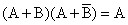.

(ii)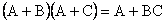.                                                                         (6)

b. What are canonical forms?                                                                                   (4)

c.  Simplify the following expression to minimum form: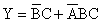.                                                                                                (6)

Q.4     a.   Find out the minimal expression for the function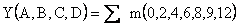.                                                             (5)

b.   Realize the following function using NAND and NOR gates only.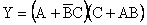(11)

Q.5     a.   What is a full adder?  Draw the circuit diagram of a 4 bit binary adder and explain its working.                                                                   (10)

b.   Use the multiplexer to implement following function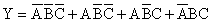(6)

Q.6     a.   Draw the circuit diagram of master slave JK flipflop and explain its working.                       (8)

b.   With the help of suitable diagram, explain the working of 4 bit parallel input serial output register.                                                               (8)

Q.7     a.   Design a mod-6 up counter, using JK flip-flops.                                                  (6)

b.   How many bits are required at the input of a ladder D/A converter, if it is required to give resolution of 5 mV and if full scale output voltage is +5V?   Draw the circuit of the DAC, Find the %age resolution.                                                                                     (10)

Q.8     a.   Explain working of dual slope A/D converter.                                                     (8)

b.   Explain the operation of 8:1 multiplexer.                                                              (8)

Q.9     a.   Explain how EPROM memory cell works.                                                        (11)

b.   A microprocessor uses RAM chips of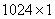capacity.

(i)                  How many chips will be required and how many address lines will be connected to provide capacity of 1024 bytes.

(ii)                How many chips will be required to obtain a memory of capacity of 16 K bytes.     (5)